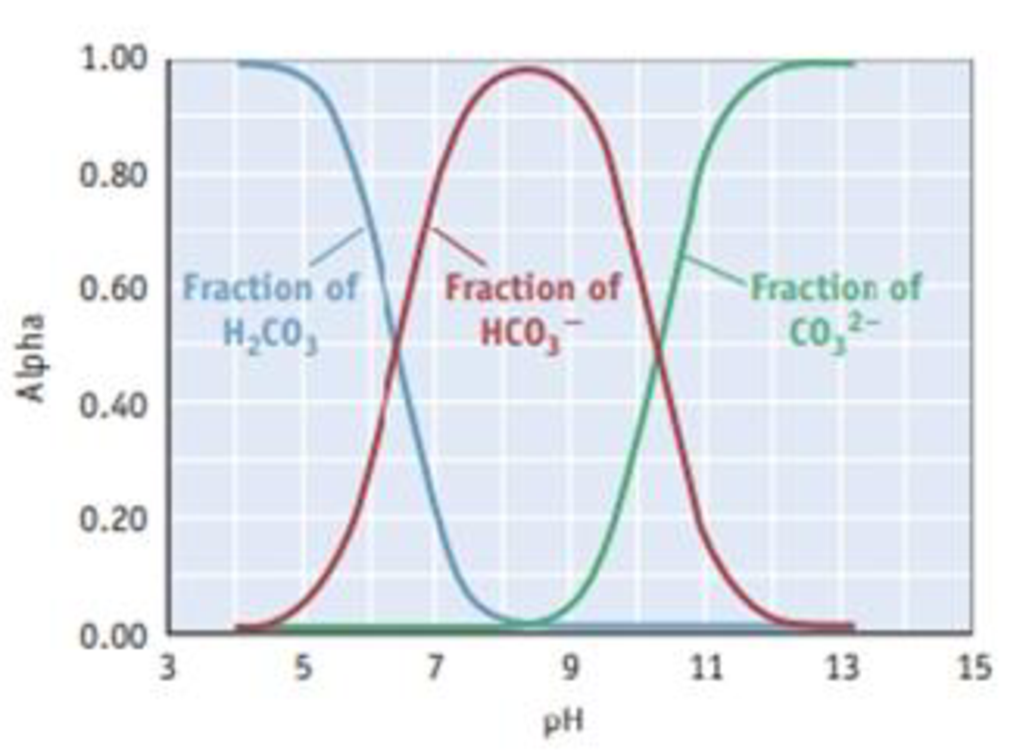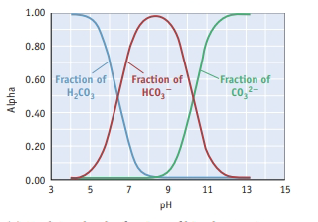Chapter 17, Problem 118SCQ

Chapter
Section
Textbook Problem

The composition diagram, or alpha plot, for the important acid-base system of carbonic acid, H2CO3, is illustrated. (See Study Question 1.7 for more information on such diagrams.)(a) Explain why the fraction of bicarbonate ion, HCO3−, rises and then falls as the pH increases. (b) What is the composition of the solution when the pH is 6.0? When the pH is 10.0? (c) If you wanted to buffer a solution at a pH of 11.0, what should be the ratio of HCO3− to CO32−?

a)

Interpretation Introduction

Interpretation:

The following by using the graph has to be determined.The fraction of bicarbonate ion, HCO3-, rises and then  falls as the pH increases has to be explained.

Concept introduction:

The pH is the unit of measurement for determining the acidity or alkalinity of a solution.

The mathematical definition of pH I negative logarithm of the molar hydrogen ion concentration is pH = -log[H+].

Explanation

At very low pH levels, [H3O+] I very high. According to  Le Chateliers   the dissociation of H2CO3 is not favourable.  As pH increases, [H3O+] decreases , causing dissociation equilibrium of H2CO3 to favour the products . It makes the [HCO3-] increases initially.

As pH increases further, [H3O+] decrease even more

b)

Interpretation Introduction

Interpretation:

The following by using the graph has to be determined.1. (a) What is the composition of the solution when the pH is 6.0 and when the pH is 10.0.

c)

Interpretation Introduction

Interpretation:

The following by using the graph has to be determined.If you wanted to buffer a solution at a pH  of 11.0, the ratio of HCO3- to CO32- has to be predicted.

Still sussing out bartleby?

Check out a sample textbook solution.

See a sample solution

The Solution to Your Study Problems

Bartleby provides explanations to thousands of textbook problems written by our experts, many with advanced degrees!

Get Started